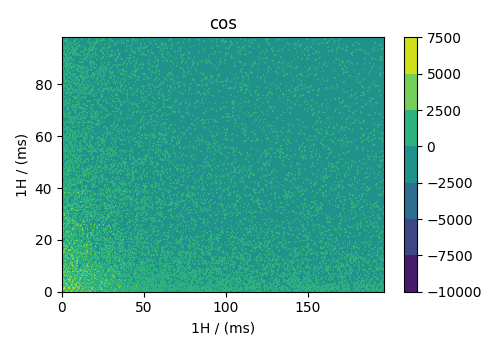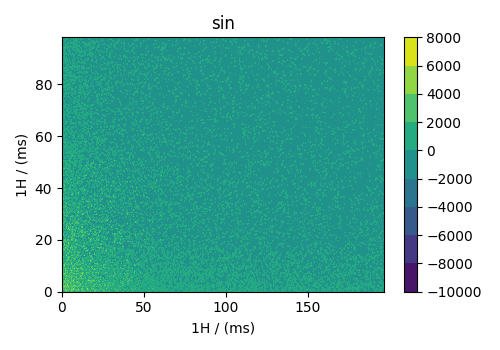Sparse along two dimensions, 2D{1,1} dataset¶

The following is an example 1 of a 2D{1,1} sparse dataset with two-dimensions, $$d=2$$, and two, $$p=2$$, sparse single-component dependent-variables, where the component is sparsely sampled along two dimensions. The following is an example of a hypercomplex acquisition of the NMR dataset.

Let’s import the CSD model data-file and look at its data structure.

import csdmpy as cp

filename = "https://osu.box.com/shared/static/kaos28g47brtswi6mgsgaap5qlahp1zo.csdf"

There are two linear dimensions and two single-component sparse dependent variables. The tuple of the dimension and the dependent variable instances are

The coordinates, viewed only for the first ten coordinates, are

print(x.coordinates[:10])

Out:

[   0.  192.  384.  576.  768.  960. 1152. 1344. 1536. 1728.] us
print(x.coordinates[:10])

Out:

[   0.  192.  384.  576.  768.  960. 1152. 1344. 1536. 1728.] us

Converting the coordinates to ms.

x.to("ms")
x.to("ms")

Visualize the dataset

import matplotlib.pyplot as plt

# split the CSDM object with two dependent variables into two CSDM objects with single
# dependent variables.

cos, sin = sparse_2d.split()

# cosine data
plt.figure(figsize=(5, 3.5))
ax = plt.subplot(projection="csdm")
cb = ax.contourf(cos.real)
plt.colorbar(cb)
plt.tight_layout()
plt.show()# sine data
plt.figure(figsize=(5, 3.5))
ax = plt.subplot(projection="csdm")
cb = ax.contourf(sin.real)
plt.colorbar(cb)
plt.tight_layout()
plt.show()Citation

1

Balsgart NM, Vosegaard T., Fast Forward Maximum entropy reconstruction of sparsely sampled data., J Magn Reson. 2012, 223, 164-169. doi: 10.1016/j.jmr.2012.07.002

Total running time of the script: ( 0 minutes 2.494 seconds)

Gallery generated by Sphinx-Gallery